###### Recent Posts

Are you interested in making your own custom animation in AR?

##### Machine Learning with Titanic Dataset
person Aman     access_time 9 months, 3 weeks ago

Problem Statement

In this blog post, we will do a complete analysis in order to determine which group of people were most likely to survive in the infamous Titanic incident. In particular, we will apply the tools of machine learning to predict which passengers would have survived the tragedy.

Detailed code for the model with more visualizations and code for this tutorial is given at this Page.

Dataset Description

The sinking of the RMS Titanic is one of the most infamous shipwrecks in history.  On April 15, 1912, during her maiden voyage, the Titanic sank after colliding with an iceberg, killing 1502 out of 2224 passengers and crew members. This sensational tragedy shocked the international community and eventually, it led to better safety regulations for ships.

The dataset is useful for those who have started learning data visualization and machine learning. We will be using Python as our working language.

Importing the necessary libraries

Here is a brief description of the libraries that we would be using:

• Numpy: it is used to perform numerical calculations in Python.

• Pandas: it is used to store data in an organized manner and to quickly manipulate it.

• Matplotlib: it is used to plot data in the form of graphs/charts for visualization.

• Seaborn: it is a Python data visualization library based on Matplotlib

• Sklearn: This library contains a lot of efficient tools for machine learning and statistical modelling including classification, regression, clustering and dimensionality reduction.

• train_test_split: IT splits arrays or matrices into a random train and test subsets

• LogisticRegression, KNeighborsClassifier, AdaBoostClassifier: Machine Learning algorithms

``````# linear algebra
import numpy as np

# data processing
import pandas as pd

# data visualization
import matplotlib.pyplot as plt
import seaborn as sns

# Algorithms
from sklearn.model_selection import train_test_split
from sklearn.linear_model import LogisticRegression
from sklearn.metrics import accuracy_score
from sklearn.neighbors import KNeighborsClassifier

Import dataset

``titanic = pd.read_csv("train.csv")``

``titanic.info()``As we can see from both the info sections, roughly 20 percent of the age data is missing. The proportion of the missing age data is small enough to be imputed without causing any major bias in the dataset. Looking at the Cabin column, it seems like we are missing too much of that data to do something useful, even at a basic level. We will either remove the cabin column or give tags like 0/1 to the values in the column.

Let’s do some Exploratory Data Analysis

As the problem statement is to predict who survived and who didn’t, let’s see what is the ratio of survived v/s not survived. We will check it out normally and then using some conditions like gender and class.

``````#people who survived v/s who didn't
sns.set_style('whitegrid')
sns.countplot(x='Survived', data= titanic, palette='RdBu_r')``````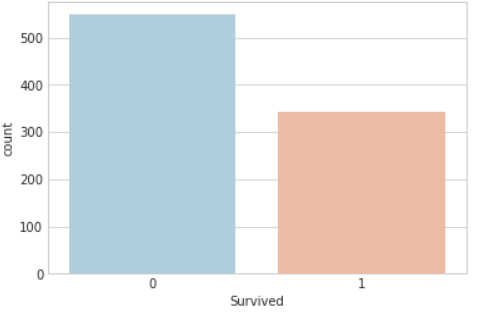``sns.countplot(x='Survived', hue='Sex', data= titanic,palette='RdBu_r')``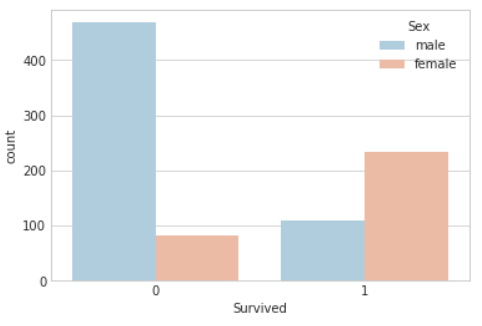It is clear that the number of survivors was significantly lower than the number of people who didn’t survive. The second plot shows that the number of males was much higher in the list of people who didn’t survive. We can observe that the number of females was significantly greater than males in the list of survivors. Maybe females first policy was used by the ship crew while transferring people on lifeboats.

``sns.countplot(x='Survived', hue='Pclass', data= titanic, palette='rainbow')``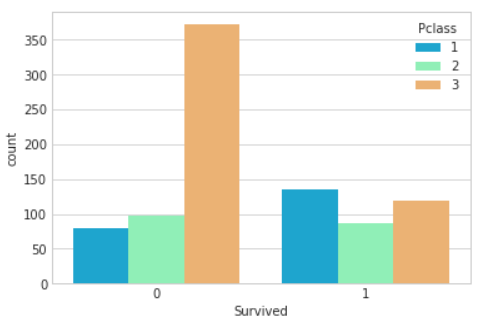The plot shows that most of the passengers who lost their lives in this tragic incident belonged to Class 3. There were more survivors from Class 1 than any other class.

Let’s plot a distribution on ‘Age’ column.

``sns.distplot(titanic['Age'].dropna(),color='darkred',bins=30)``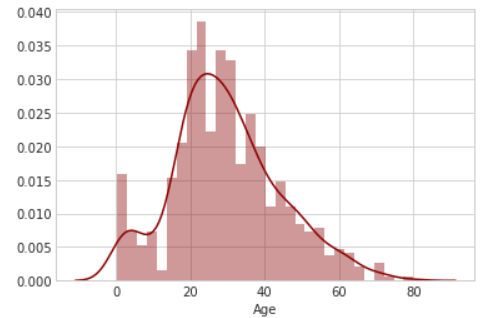The mean age of people on board was 29-30 with a standard deviation of 14. The distribution for age is slightly right-skewed.

``titanic['Fare'].hist(color='green',bins=40,figsize=(8,4))``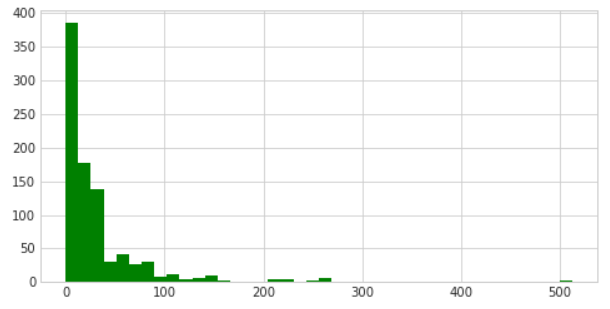The ‘Fare’ column is highly right-skewed with a few outliers.

Data Cleaning

Let’s do some data cleaning. We have to fill in the missing values in the age column and then take care of the ‘Cabin’ column.

Let's fill in the missing values of the age column. We can do this by taking the mean of the age. The smarter way will be to fill in the missing blanks with the mean of the Pclass they belong to.

``````plt.figure(figsize=(12, 7))
sns.boxplot(x='Pclass',y='Age',data=titanic,palette='winter')``````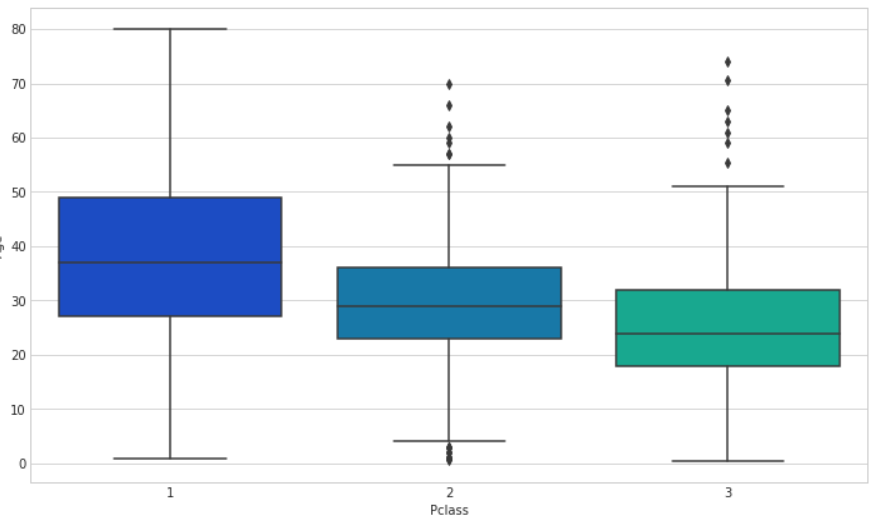We can see that the wealthier passengers in the higher classes tend to be older, which makes sense. We’ll use these average age values to impute based on Pclass for Age.

``````def impute_age(cols):
Age = cols
Pclass = cols
if pd.isnull(Age):
if Pclass == 1:
return 37
elif Pclass == 2:
return 29
else:
return 24
else:
return Age

titanic['Age'] = titanic[['Age', 'Pclass']].apply(impute_age, axis = 1)``````

Let’s go ahead and give 1 tag to value with valid Cabin no. and 0 tags to value with NaN.

``````def impute_cabin(col):
Cabin = col
if type(Cabin) == str:
return 1
else:
return 0

titanic['Cabin'] = titanic[['Cabin']].apply(impute_cabin, axis = 1)``````

We are finished dealing with the ‘NaN’ values in the dataset. Let’s check the head of the dataset.We’ll need to convert categorical features to dummy variables using pandas! Otherwise, our machine learning algorithm won’t be able to directly take in those features as inputs.

``````#Let's work on a copy of our present dataset for further operations
dataset = titanic

sex = pd.get_dummies(dataset['Sex'],drop_first=True)
embark = pd.get_dummies(dataset['Embarked'],drop_first=True)
dataset.drop(['Sex','Embarked','Name','Ticket'],axis=1,inplace=True)
dataset = pd.concat([dataset,sex,embark],axis=1)``````

Following is our new cleaned dataset on which we will be applying our machine learning models.

``dataset.head()``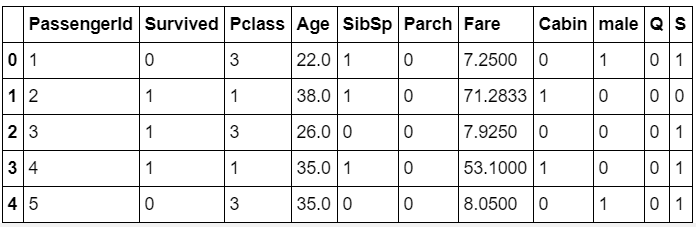Let’s do some Machine Learning

We will be using:

• ?• Logistic Regression

• ?• SVM

To train our model.

Why are we using these ML algorithms?

Logistic regression is the most basic classification algorithm. It is a variant of a linear regression which is used for solving regression problems. Despite being a basic algorithm, it’s capable of catching the variations in the training dataset when the dependent variable is binary. As for the surviving feature we are dealing with only 1 and 0, so it will provide us with some great insights.

Support Vector Machines also perform well when the dependent variable is binary. The data value lies on one side of the two support vectors. Plus, the advantage of using SVM is that it is not affected by outliers. There were some outliers in the fare feature of our dataset so we will give it a try.

AdaBoost is one of the best-boosting algorithms we have. It works efficiently when the dataset is large enough. We will use it just to test how much a big fish is capable of surviving when there is not enough food.

#### Prepare data for training and testing

``````#Train Test Split
X_train, X_test, y_train, y_test = train_test_split(dataset.drop('Survived',axis=1),dataset['Survived'], test_size=0.25,random_state=101)``````

#### Logistic Regression

``````regressor = LogisticRegression()
regressor.fit(X_train, y_train)
pred = regressor.predict(X_test)

Let’s evaluate our results on the X_test part of the dataset.

print(accuracy_score(y_test, pred))

0.816143497758``````

Hmmm… An accuracy of approximately 82% is not that bad when the training set was not large enough. Maybe we can improve the accuracy using some feature engineering. But that is for another blog post. Let’s train the dataset using more classification models.

#### SVM

``````regressor2 = SVC()
regressor2.fit(X_train, y_train)

pred2 = regressor2.predict(X_test)
print(accuracy_score(y_test, pred2))

0.600896860987``````

Clearly, SVM gives very less accuracy on this dataset. As the no. of features in the dataset are more, SVM performs less efficiently. What can we do to increase accuracy? The kernel parameter used by default in SVM is linear. We change it to “Gaussian” and can retrain the model accordingly.

``````regressor4 = AdaBoostClassifier()
regressor4.fit(X_train, y_train)

pred4 = regressor4.predict(X_test)
print(accuracy_score(y_test, pred4))

0.807174887892``````

By using this, we again obtained a good accuracy of 80.7%.

We have shown you how to approach a dataset by exploring it using Exploratory Data Analysis and then building the model to predict the results.

Keep Learning!!!

Hopefully, this was helpful and don’t hesitate to let us know if any of those steps don’t work anymore or if anything seems confusing! Post your doubts in the comments section.

You may also be interested in reading the following: How do I learn Machine Learning?Machine Learning with Iris Dataset and Machine Learning Roadmap